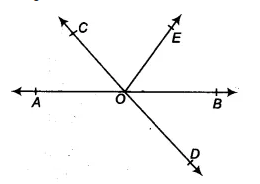Q

# In Fig. 6.13, lines AB and CD intersect at O. If ∠ AOC + ∠ BOE = 70° and ∠ BOD = 40°, find ∠ BOE and reflex ∠ COE.

1. In Fig. 6.13, lines AB and CD intersect at O. If AOC + BOE = 70° and BOD = 40°, find BOE and reflex COE.ViewsGiven that,
AB is a straight line. Lines AB and CD intersect at O.  and  BOD =
Since AB is a straight line
AOC + COE + EOB =
[since ]

So, reflex COE =
It is given that AB and CD intersect at O
Therefore, AOC  = BOD  [vertically opposite angle]
[ GIven  BOD = ]
Also,
So,  BOE =

Exams
Articles
Questions